Next: Completeness Up: Klein-Gordon Equation Previous: Coulomb Interaction

# Homogeneous Green Function

If we know the wave function at time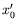, then it is give at some other time,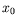, using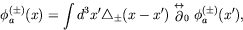(4.170)

where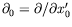and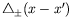is a Green function.

Because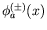satisfies Klein-Gordon equation, so does the Green function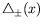: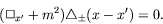(4.171)

The most general solution with this property is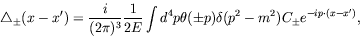(4.172)

where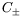is a scalar function of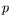alone. Thereforeis a constant as it must be a function of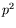only, which is a constant. To see this, we use plane waves with momentum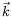and write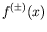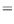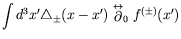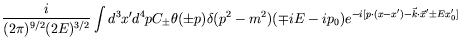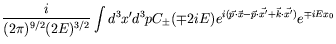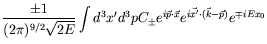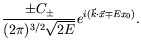(4.173)

Therefore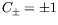.

It is also useful to write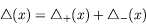(4.174)

so that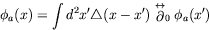(4.175)

is the Green theorem for any solution of the Klein-Gordon equation.

Since the Schrödinger equation is first order, this means that given the state vector at time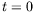, one can determine the state at all future times. On the other hand, since the Klein-Gordon equation is second order, one requires two initial conditions - both the wave function and its time derivative. These problems are resolved by the realization that a properly relativistic wave equation involves of necessity both particle and antiparticle degrees of freedom. The existence of both particle and antiparticle degrees of freedom in the wave function is the reason that one needs two boundary conditions at timein order to predict the future behaviour.Next: Completeness Up: Klein-Gordon Equation Previous: Coulomb Interaction
Douglas M. Gingrich (gingrich@ ualberta.ca)
2004-03-18